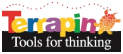Terrapin Resources

# String Art

by jonesv@ccsu.eduInspired by the mathematical research of Harlan Brothers at the Country School in Madison, CT, Prof. Virginia Jones at Central Connecticut State University created Terrapin Logo procedures to show Koch string variations. Email Prof. Jones at jonesv@ccsu.edu for a document explaining the mathematics behind the calculations.

This project illustrates Koch string variations.

#### KochStrings.lgo

``````  to koch_hb :length :level :theta
; This procedure was created by Harlan Brothers, 2003.
if (:level = 0) [fd :length stop]
koch_hb  (:length/(2*(1 + cos (:theta)))) (:level - 1) :theta
lt :theta
koch_hb  (:length/(2*(1 + cos (:theta)))) (:level - 1) :theta
rt 2* :theta
koch_hb  (:length/(2*(1 + cos (:theta)))) (:level - 1) :theta
lt :theta
koch_hb  (:length/(2*(1 + cos (:theta)))) (:level - 1) :theta
end

to string
; This procedure was created by Virginia Jones, 2003.
; If you would like a word document explaining the math
; behind the calculation for :length/(2*(1 + cos (:theta)))
; please email Virginia Jones at:  jonesv@ccsu.edu
; This creates a horizontal string "centered"
; in the full-screen graphics window with the "tip" of the
; string 8 pixels below the home position.
; To create a string from a current position of the turtle
; use the procedure koch_string by itself
; you can change the 198 assigned to :base
; which gives half the length of the string base
make "base1 198
cs fs pu rt 90 bk :base1 lt 90 bk :base1 rt 90 pd
koch_string :base1 * 2
end

to koch_string :base2
; you can change the variation in the angle theta
; by assigning a value other than 88 in the following make command
; and subtracting a number other than 4 in the make command
; in the repeat line
; WARNING you will then need to "adjust" the repeat value of 23 to
; an appropriate new number
make "theta 88
repeat 23 [ koch_hb :base2 1 :theta make "theta :theta - 4  pu bk :base2 pd]
end
``````

 Procedure STRING Description Koch string variations Level Intermediate Tags Koch, Strings, Formulae